# 1617-257/TUT-R-8

Problem 1. Let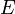$E$ be an infinite subset of a compact metric space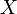$X$. Show that$E$ has a limit point.
Proof. If$E$ has no limit points, then$E$ is a closed subset of a compact space and is therefore compact in itself. Since each point of$E$ is isolated, we may find for each point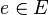$e \in E$ a neighborhood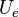$U_e$ such that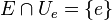$E \cap U_e = \{ e \}$. The collection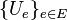$\{ U_e\}_{e \in E}$ is an open cover of E which clearly has no finite subcover.
Problem 2. Let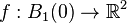$f: B_1(0) \to \mathbb{R}^2$ be a function which is "jelly-rigid": for all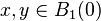$x,y \in B_1(0)$: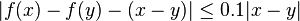$|f(x) - f(y) - (x - y)| \leq 0.1 |x - y|$. Prove that$f$ maps onto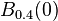$B_{0.4}(0)$.
Proof. Since$f$ is Lipschitz, it has a unique extension to its boundary and so we regard it as a function on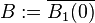$B:= \overline{B_1(0)}$. A simple estimate shows if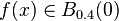$f(x) \in B_{0.4}(0)$, then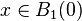$x \in B_1(0)$. Suppose now that there is some point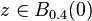$z \in B_{0.4}(0)$ which is not in the image of$f$. Let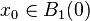$x_0 \in B_1(0)$ be a closest element to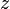$z$ in the image of$f$. Consider now the point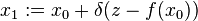$x_1 := x_0 + \delta (z - f(x_0))$ (jelly-rigidity says that the function is almost like the identity, so moving closer to the point$z$ from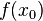$f(x_0)$ in the codomain side can be obtained by moving in that same direction on the domain side first) where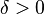$\delta > 0$ is chosen to be small enough so that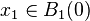$x_1 \in B_1(0)$ and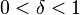$0< \delta < 1$. Then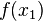$f(x_1)$ is closer to$z$ than is$f(x_0)$.